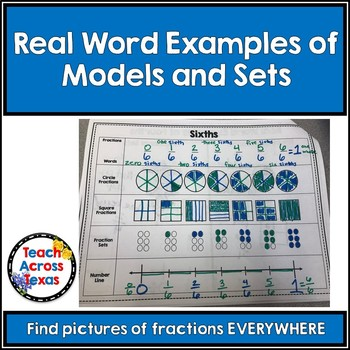# My Fraction Book about Halves, Thirds, Fourths, Sixths & Eighths 3.3A 3.3BSubject
Grade Levels
Resource Type
Product Rating
File Type

PDF (Acrobat) Document File

Be sure that you have an application to open this file type before downloading and/or purchasing.

1 MB
Share
Product Description

This is a fraction book for kids. It has halves, thirds, fourths, sixths and eighths. It has the following representations for each one: Fractions, Fraction words, circle models, square models, fraction sets, and a number line. Each one is lined up so students can see what one fraction would look like on each representation. I use this to introduce fractions when I am teaching TEKS 3.3A and 3.3B. There is a Real Word Examples page for each denominator, students can find real world examples of both models and fraction sets. I have included some examples at the end. This is a fun way to let students explore the many representation of fractions. I have included at page with real word examples of both fraction models and fraction sets. I usually print these out and have them available in case some friends can not do the examples at home. A tip when writing fraction words and the fraction in this book: I have my students write the numerator in a certain color and the denominator in a certain color. Then when we are writing the fraction words they write the numerator in its color and denominator in its color to help them learn how to write a fraction in words.

Total Pages
N/A
Answer Key
N/A
Teaching Duration
N/A
Report this ResourceSign Up